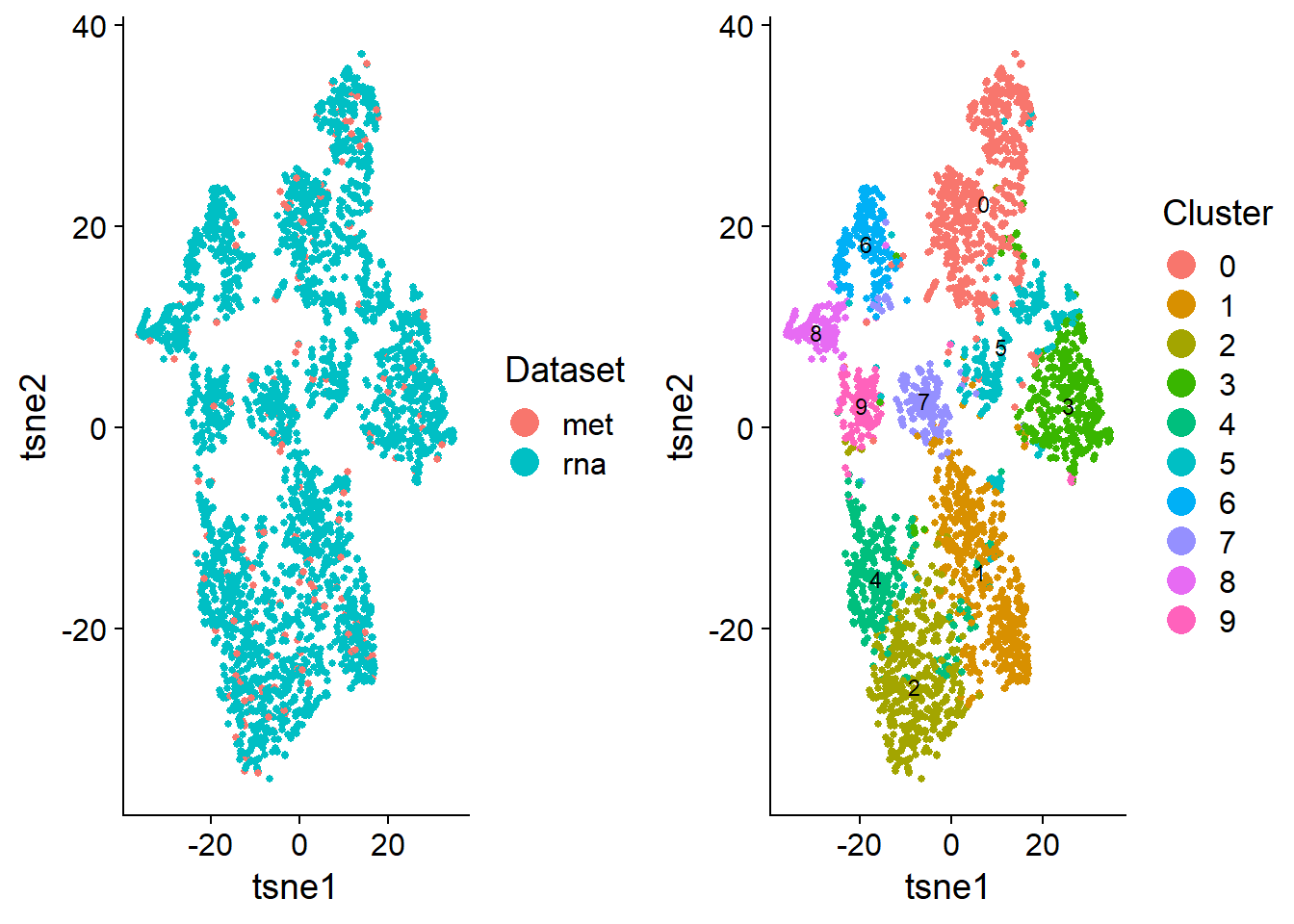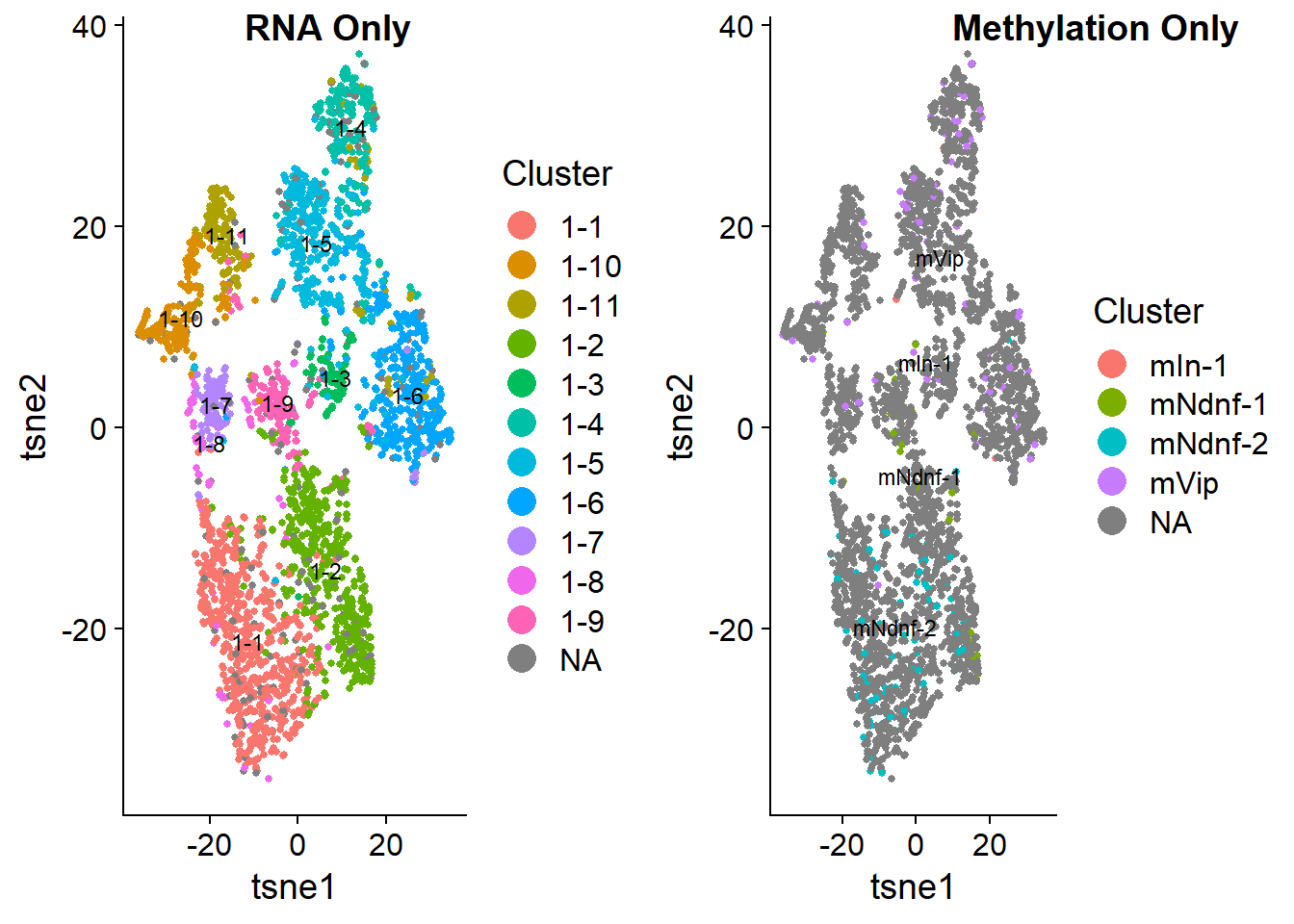## Introduction

This vignette shows how to use LIGER to jointly define cell types from single-cell gene expression and DNA methylation. We will be using scRNA-seq and single-nucleus DNA methylation data from mouse cortex. These are the same datasets used in our recent paper. The steps involved are quite similar to those for integrating multiple RNA datasets. The only differences are (1) we process the methylation data into gene-level values comparable to gene expression, (2) we perform gene selection using the RNA data only, and (3) we both scale and center the factor loadings before performing quantile normalization.

The full dataset used in the paper is quite large, so in this example, we restrict our attention to inhibitory interneurons derived from the caudal ganglionic eminence (CGE). We downloaded the gene-level methylation data provided by the Ecker lab. They generated these values by dividing the numbers of methylated (non-CG methylation, mCH) and detected positions across the gene body within each cell. They and we found these gene body mCH proportions to be negatively correlated with gene expression level in neurons. The gene expression data is from Saunders et al. 2018. The full dataset is available for download through Dropviz. For convenience, we provide the cells x genes count matrices for only CGE interneurons here.

``````rna <- readRDS('mouse_frontal_cortex_cge_rna.RDS')

## Create liger object and preprocess data

We then create a liger object using the methylation and expression data. The selectGenes function performs variable gene selection on each of the datasets separately, then takes the union of the result. The variable genes are selected by comparing the variance of each gene’s expression to its mean expression. The selectGenes function was written primarily scRNA-seq in mind, and the methylation data distribution is quite different. So instead of taking the union of variable genes from RNA and methylation, we set datasets.use = 1 in the function to perform gene selection using only the RNA dataset. Because gene body mCH proportions are negatively correlated with gene expression level in neurons, we need to reverse the direction of the methylation data. We do this by simply subtracting all values from the maximum methylation value. The resulting values are positively correlated with gene expression. In addition, the proportional nature of the gene body methylation makes it unnecessary to normalize and scale the methylation data. Thus, we simply overwrite the scaled methylation data with the reversed methylation data.

``````library(liger)
rna.met <- createLiger(list(rna=rna,met=met))
rna.met <- normalize(rna.met)
rna.met <- selectGenes(rna.met,datasets.use=c(1))
rna.met <- scaleNotCenter(rna.met)
rna.met@scale.data[] <- max(rna.met@raw.data[]) - t(as.matrix(rna.met@raw.data[][rna.met@var.genes,]))``````

## Factorize and perform quantile normalization

Next we perform integrative non-negative matrix factorization in order to identify shared and distinct metagenes across the datasets and the corresponding metagene loadings for each cell. The most important parameters in the factorization are `k` (the number of factors) and `lambda` (the penalty parameter which limits the dataset-specific component of the factorization). The default value of `lambda=5.0` usually provides reasonable results for most analyses. For this analysis, we simply use `k = 20` and the default value of `lambda`.

``rna.met = optimizeALS(rna.met,k=20)``

To jointly define cell types, we perform a quantile normalization step. This process first identifies similarly loading cells across datasets by building a similarity graph based on shared factor neighborhoods. Using Louvain community detection, we then jointly identify clusters across datasets, and normalize quantiles within each cluster and factor. The key parameters in this step are `resolution` (increasing this increases the number of communities detected) and `knn_k` (the number of dataset neighbors used in generating the shared factor neighborhood). In general, lowering `knn_k` will allow for more fine-grained identification of smaller groups, but possibly at the cost of decreased alignment across datasets. Here we simply use default settings of `resolution=1.0` and `knn_k=20`. The methylation data matrix is dense (due to reversing the direction of the signal by subtracting from the max), so the factor loadings will also be dense, and we need to both scale and center the loadings with the parameter `center=T`.

``rna.met = quantileAlignSNF(rna.met,center=T)``
``````##  "Recomputing shared nearest factor space"
##  "making edge file."
##  "Starting SLM"``````

## Visualize results

We run t-SNE on the normalized factors, then color the t-SNE coordinates by dataset and cluster.

``````rna.met = runTSNE(rna.met)
ggplot2::theme_set(theme_cowplot())
by_joint = plotByDatasetAndCluster(rna.met,return.plots=T,pt.size=1)
plot_grid(by_joint[],by_joint[])``````The t-SNE plot shows that the datasets align well and indicates the jointly inferred clusters. Using the original RNA and methylation cluster assignments, we can visually confirm that the joint analysis is highly consistent with the single-modality analyses.

``````by_rna = plotByDatasetAndCluster(rna.met,clusters=rna_clusts,return.plots=T,pt.size=1)
by_met = plotByDatasetAndCluster(rna.met,clusters=met_clusts,return.plots=T,pt.size=1)
plot_grid(by_rna[],by_met[],labels = c("RNA Only","Methylation Only"),label_x=0.25)``````Plotting marker genes for subtypes of CGE interneurons confirms that the data types are properly aligned, with the expected inverse relationship between gene body mCH and gene expression.

``````vip = plotGene(rna.met,gene="Vip",return.plots=T,pt.size=1)
lamp5 = plotGene(rna.met,gene="Lamp5",return.plots=T,pt.size=1)
plot_grid(vip[],lamp5[],vip[],lamp5[],nrow = 2)``````